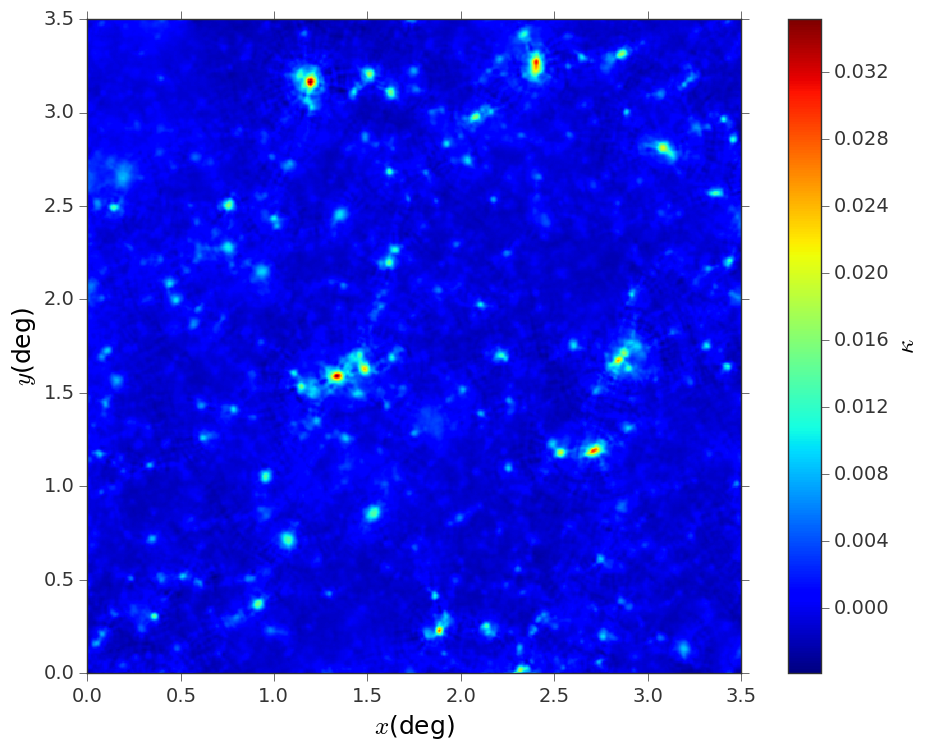Construct convergence maps out of shear catalogs¶

Constructing a convergence map of a particular subfield from a shear catalog is an operation that occurs frequently in weak lensing analysis; if the field of view is unmasked, the reconstruction operation is equivalent to the calculation of the $$E$$ mode of the shear field $$\gamma$$. Here is how to to use lenstools to perform this operation: the operations are handled with the ShearCatalog class

from lenstools.catalog.shear import ShearCatalog
import astropy.table as tbl
import astropy.units as u

Suppose that the shear table is contained in a file called ‘WLshear.fits’ (which should contain a table with columns ‘shear1’,’shear2’ readable with astropy.table.Table.read()), in which each row represents a different galaxy; suppose also that the sky positioning information $$(x,y)$$ is contained in a file ‘positions.fits’; we must combine the information in these two tables to build a $$(x,y,\gamma)$$ table

convergence_map.visualize(colorbar=True,cbar_label=r"$\kappa$")